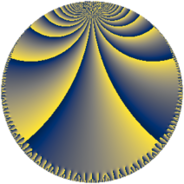# Properties

 Label 192.10.sLevel $192$ Weight $10$ Character orbit 192.s Rep. character $\chi_{192}(11,\cdot)$ Character field $\Q(\zeta_{16})$ Dimension $2288$ Sturm bound $320$

# Related objects

## Defining parameters

 Level: $$N$$ $$=$$ $$192 = 2^{6} \cdot 3$$ Weight: $$k$$ $$=$$ $$10$$ Character orbit: $$[\chi]$$ $$=$$ 192.s (of order $$16$$ and degree $$8$$) Character conductor: $$\operatorname{cond}(\chi)$$ $$=$$ $$192$$ Character field: $$\Q(\zeta_{16})$$ Sturm bound: $$320$$

## Dimensions

The following table gives the dimensions of various subspaces of $$M_{10}(192, [\chi])$$.

Total New Old
Modular forms 2320 2320 0
Cusp forms 2288 2288 0
Eisenstein series 32 32 0

## Trace form

 $$2288 q - 8 q^{3} - 16 q^{4} - 8 q^{6} - 16 q^{7} - 8 q^{9} + O(q^{10})$$ $$2288 q - 8 q^{3} - 16 q^{4} - 8 q^{6} - 16 q^{7} - 8 q^{9} - 16 q^{10} - 8 q^{12} - 16 q^{13} - 8 q^{15} - 16 q^{16} - 8 q^{18} - 16 q^{19} - 8 q^{21} - 16 q^{22} - 12097008 q^{24} - 16 q^{25} - 8 q^{27} - 16 q^{28} - 44263288 q^{30} - 32 q^{31} - 16 q^{34} + 110500712 q^{36} - 16 q^{37} - 8 q^{39} - 16 q^{40} + 224559072 q^{42} - 16 q^{43} - 8 q^{45} - 16 q^{46} - 8 q^{48} - 16 q^{49} - 8 q^{51} + 25227488 q^{52} - 8 q^{54} - 280217680 q^{55} - 8 q^{57} - 1710306592 q^{58} - 8 q^{60} - 16 q^{61} + 1364809808 q^{64} - 4104 q^{66} + 1611351920 q^{67} - 8 q^{69} - 1869092080 q^{70} - 8 q^{72} - 16 q^{73} - 8 q^{75} + 1555244192 q^{76} + 2023944064 q^{78} + 2269128656 q^{79} - 8 q^{81} + 3136405664 q^{82} + 6148771880 q^{84} - 16 q^{85} - 8 q^{87} - 4940685376 q^{88} - 7222410008 q^{90} - 16 q^{91} - 157472 q^{93} + 6710490128 q^{94} + 10366581632 q^{96} - 8 q^{99} + O(q^{100})$$

## Decomposition of $$S_{10}^{\mathrm{new}}(192, [\chi])$$ into newform subspaces

The newforms in this space have not yet been added to the LMFDB.